Next: Three-Dimensional Wave Mechanics Up: Wave Mechanics Previous: Wavefunction Collapse

# Stationary States

Consider separable solutions to Schrödinger's equation of the form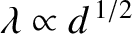(1138)

According to Equation (1099), such solutions have definite energies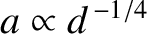. For this reason, they are usually written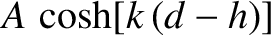(1139)

The probability of finding the particle between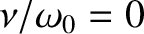and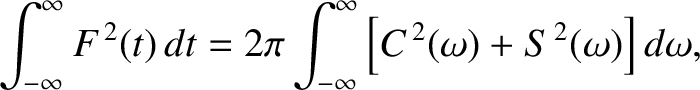at time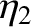is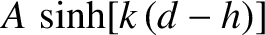(1140)

This probability is time independent. For this reason, states whose wavefunctions are of the form (1139) are known as stationary states. Moreover,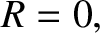is called a stationary wavefunction. Substituting (1139) into Schrödinger's equation, (1102), we obtain the following differential equation for:(1141)

This equation is called the time independent Schrödinger equation.

Consider a particle trapped in a one-dimensional square potential well, of infinite depth, which is such that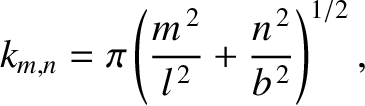(1142)

The particle is excluded from the region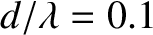or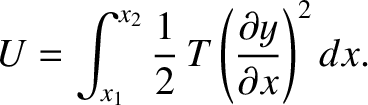, soin this region (i.e., there is zero probability of finding the particle outside the well). Within the well, a particle of definite energy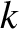has a stationary wavefunction,, that satisfies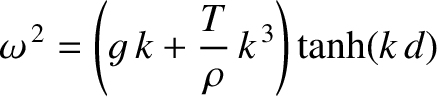(1143)

The boundary conditions are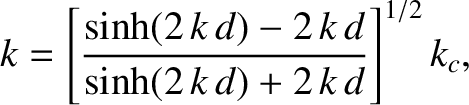(1144)

This follows becausein the regionor, andmust be continuous [because a discontinuous wavefunction would generate a singular term (i.e., the term involving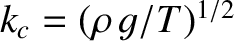) in the time independent Schrödinger equation, (1141), which could not be balanced, even by an infinite potential].

Let us search for solutions to Equation (1143) of the form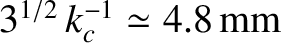(1145)

where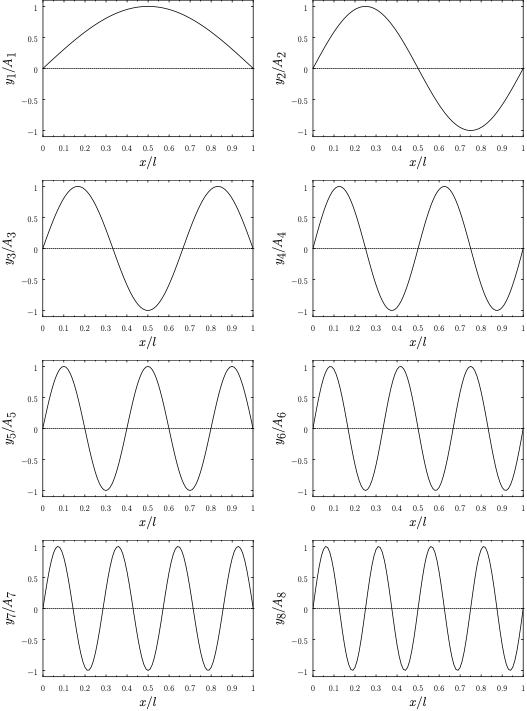is a constant. It follows that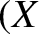(1146)

The solution (1145) automatically satisfies the boundary condition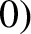. The second boundary condition,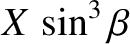, leads to a quantization of the wavenumber: that is,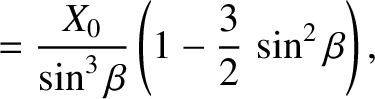(1147)

where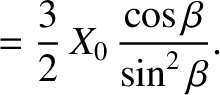et cetera. (A quantized'' quantity is one that can only take certain discrete values.) According to Equation (1146), the energy is also quantized. In fact,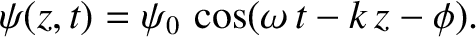, where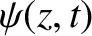(1148)

Thus, the allowed wavefunctions for a particle trapped in a one-dimensional square potential well of infinite depth are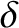(1149)

where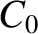is a positive integer, and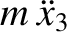a constant. We cannot have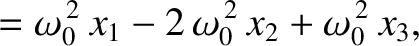, because, in this case, we obtain a null wavefunction: that is,, everywhere. Furthermore, iftakes a negative integer value then it generates exactly the same wavefunction as the corresponding positive integer value (assuming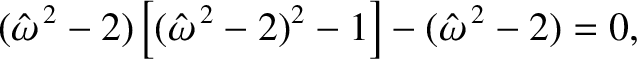).

The constant, appearing in the previous wavefunction, can be determined from the constraint that the wavefunction be properly normalized. For the case under consideration, the normalization condition (1107) reduces to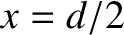(1150)

It follows from Equation (1149) that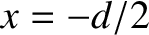. Hence, the properly normalized version of the wavefunction (1149) is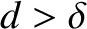(1151)

Figure 81 shows the first four properly normalized stationary wavefunctions for a particle trapped in a one-dimensional square potential well of infinite depth: that is ,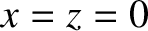, for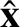to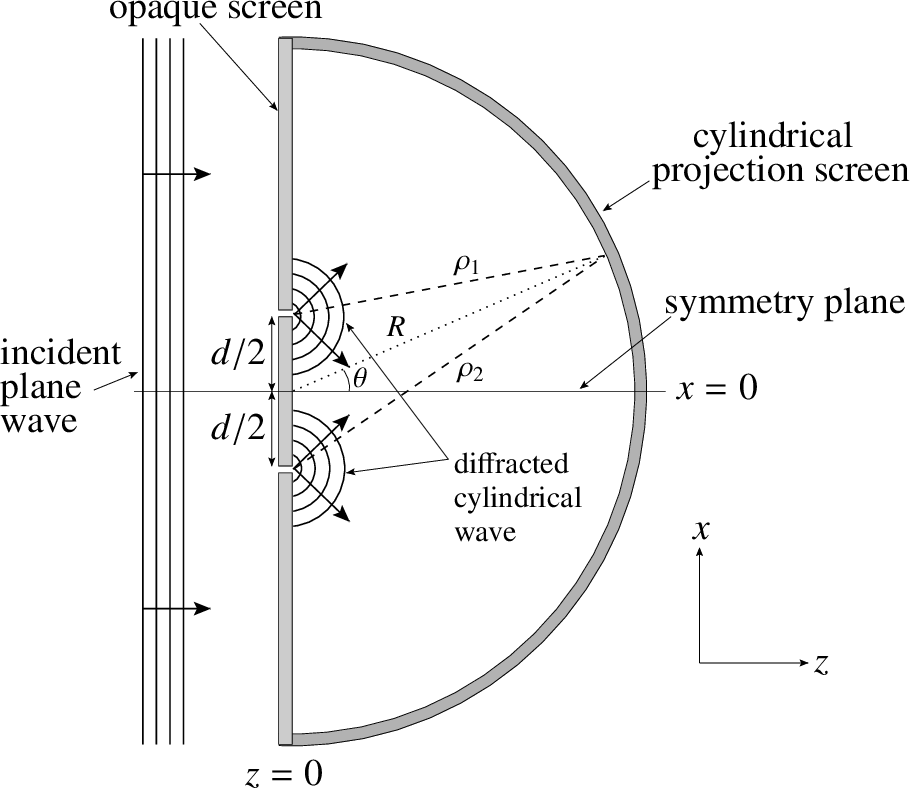.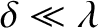The stationary wavefunctions that we have just found are, in essence, standing wave solutions to Schrödinger's equation. Indeed, the wavefunctions are very similar in form to the classical standing wave solutions discussed in Chapters 5 and 6.

At first sight, it seems rather strange that the lowest possible energy for a particle trapped in a one-dimensional potential well is not zero, as would be the case in classical mechanics, but rather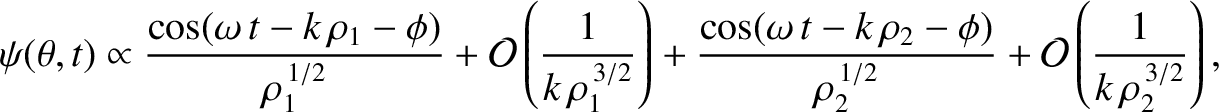. In fact, as explained in the following, this residual energy is a direct consequence of Heisenberg's uncertainty principle. A particle trapped in a one-dimensional well of width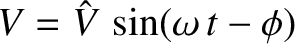is likely to be found anywhere inside the well. Thus, the uncertainty in the particle's position is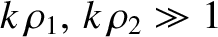. It follows from the uncertainty principle, (1135), that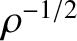(1152)

In other words, the particle cannot have zero momentum. In fact, the particle's momentum must be at least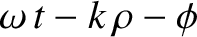. However, for a free particle,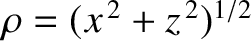. Hence, the residual energy associated with the particle's residual momentum is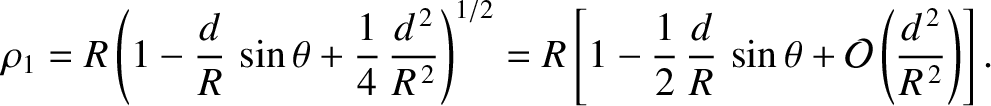(1153)

This type of residual energy, which often occurs in quantum mechanical systems, and has no equivalent in classical mechanics, is called zero point energy.Next: Three-Dimensional Wave Mechanics Up: Wave Mechanics Previous: Wavefunction Collapse
Richard Fitzpatrick 2013-04-08